## 1. Introduction

In this tutorial, we’ll learn about Radix Sort, analyze its performance, and take a look at its implementation.

Here we focus on using Radix Sort to sort integers, but it’s not limited to just numbers. We can use it to sort other types such as String, too.

In order to keep it simple, we’re gonna focus on the decimal system in which the numbers are expressed in base (radix) 10.

## 2. Algorithm Overview

Radix sort is a sorting algorithm that sorts numbers based on the positions of their digits. Basically, it uses the place value of the digits in a number. Unlike most of the other sorting algorithms, such as Merge Sort, Insertion Sort, Bubble Sort, it doesn’t compare the numbers.

Radix sort uses a stable sorting algorithm as a subroutine to sort the digits. We’ve used a variation of counting sort as a subroutine here that uses the radix to sort the digits in every position. Counting sort is a stable sorting algorithm and it works well in practice.

Radix sort works by sorting digits from the Least Significant Digit (LSD) to the Most Significant Digit (MSD). We can also implement Radix sort to process digits from MSD.

## 3. A Quick Example

Let’s see how it works with an example. Let’s consider the following array: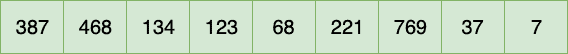### 3.1. Iteration 1

We’ll sort this array by processing digits from LSD and moving towards MSD.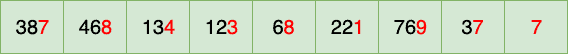After the first iteration, the array now looks like: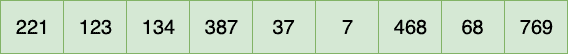Note that the numbers have been sorted according to the digits in ones place.

### 3.2. Iteration 2

Let’s move on to the digits in tens place: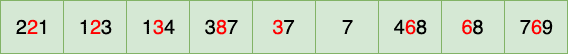Now the array looks like: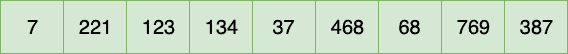We see that the number 7 has occupied the first position in the array since it doesn’t have any digit in the tens place. We could also think of this as having a 0 in the tens place.

### 3.3. Iteration 3

Let’s move on to the digits in the hundreds position: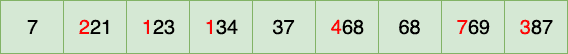After this iteration, the array looks like: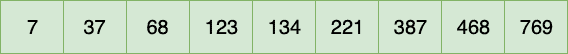And the algorithm stops here, with all elements sorted.

## 4. Implementation

Let’s now look at the implementation.

``````void sort(int[] numbers) {
int maximumNumber = findMaximumNumberIn(numbers);
int numberOfDigits = calculateNumberOfDigitsIn(maximumNumber);
int placeValue = 1;
while (numberOfDigits-- > 0) {
applyCountingSortOn(numbers, placeValue);
placeValue *= 10;
}
}``````

The algorithm works by finding out the maximum number in the array and then calculating its length. This step helps us to ensure that we execute the subroutine for every place value.

For example, in the array, [7, 37, 68, 123, 134, 221, 387, 468, 769], the maximum number is 769 and its length is 3.

So, we iterate and apply the subroutine thrice on the digits in every position:

``````void applyCountingSortOn(int[] numbers, int placeValue) {

int range = 10 // decimal system, numbers from 0-9

// ...

// calculate the frequency of digits
for (int i = 0; i < length; i++) {
int digit = (numbers[i] / placeValue) % range;
frequency[digit]++;
}

for (int i = 1; i < range; i++) {
frequency[i] += frequency[i - 1];
}

for (int i = length - 1; i >= 0; i--) {
int digit = (numbers[i] / placeValue) % range;
sortedValues[frequency[digit] - 1] = numbers[i];
frequency[digit]--;
}

System.arraycopy(result, 0, numbers, 0, length);

}``````

In the subroutine, we’ve used the radix (range) to count the occurrence of each digit and increment its frequency. So, each bin in the range from 0 to 9 will have some value based on the frequency of digits. We then use the frequency to position each element in the array. This also helps us to minimize the space required to sort the array.

Now let’s test our method:

``````@Test
int[] numbers = {387, 468, 134, 123, 68, 221, 769, 37, 7};
int[] numbersSorted = {7, 37, 68, 123, 134, 221, 387, 468, 769};
}``````

## 5. Radix Sort vs Counting Sort

In the subroutine, the length of the frequency array is 10 (0-9). In the case of Counting Sort, we don’t use the range. The length of the frequency array will be the maximum number in the array + 1. So we don’t divide them into bins whereas Radix Sort uses the bins to sort.

Counting Sort is quite efficient when the length of the array is not much smaller than the maximum value in the array whereas Radix Sort allows for larger values in the array.

## 6. Complexity

The performance of Radix Sort depends on the stable sorting algorithm chosen to sort the digits.

Here we’ve used the Radix Sort to sort an array of n numbers in base b. In our case, the base is 10. We’ve applied the Counting Sort d times where d stands for the number of digits. So the time complexity of Radix Sort becomes O(d * (n + b)).

The space complexity is O(n + b) since we have used a variation of Counting Sort as a subroutine here.